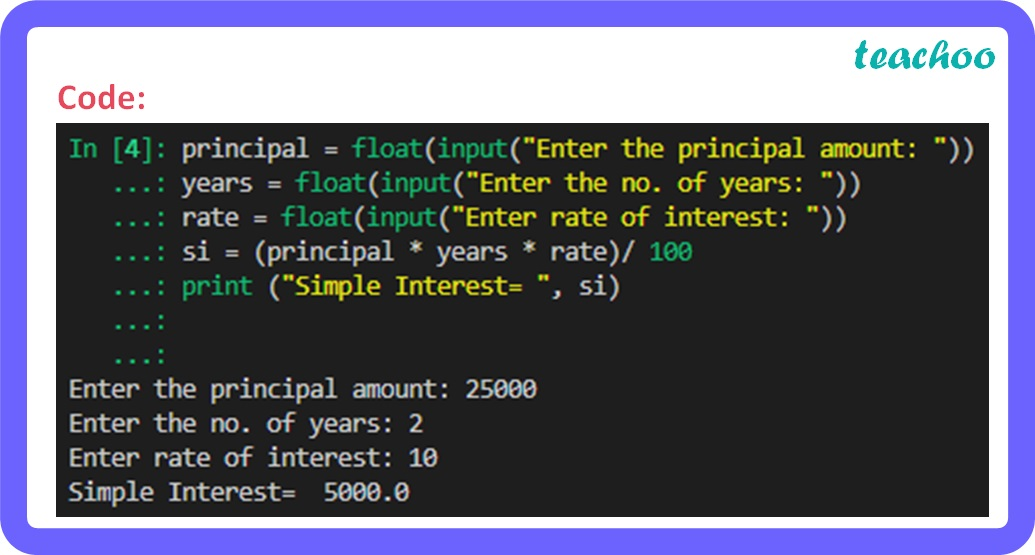Short Answer Type Questions (2 Marks each)

Computer Science - Class 11
Chapter 5 Class 11 - Getting started with python

## Write a program to calculate simple interest.

Code:

``` principal = float(input("Enter the principal amount: "))  ```

``` years = float(input("Enter the no. of years: "))  ```

``` rate = float(input("Enter rate of interest: "))  ```

``` si = (principal * years * rate)/ 100  ```

``` print ("Simple Interest= ", si) ```Learn in your speed, with individual attention - Teachoo Maths 1-on-1 Class